Next: The Central Problem of Up: text_knot Previous: What is a "mathematical"

# Definitions

• Knot - A knot is a simple closed curve in 3-dimensional space. Note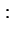Deforming a knot does not change it.What does that mean? Well, a loop like the one above is considered a knot in mathematical knot theory (it is a simple closed curve in 3-dimensional space). In fact this knot has a special name: The unknot. The unknot can be drawn with no crossings, and is also called a trivial knot. It is the simplest of all knots.

• Projection - Different ways of projecting a knot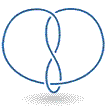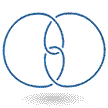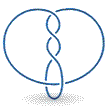Three projections of the figure-eight knot

• Crossing - The places where the knot crosses itself NoteThe figure eight knot is a four crossing knot.

• Topology - It is the study of the geometric objects and their properties

• Alternating Knot - It is a knot with a projection that has crossing that alternate between over and under as one travels around the knot in a fixed direction.

• Composition of Knots - When an arc is cut from two projections of knots and the four ends are connected by two new arcs, the resulting knot is called the composition of two knots (J) & (K), represented by (J # K).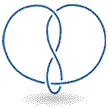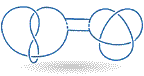• Composite Knot - It is a knot the can be expressed as the composition of two knots (not unknots).

• Factor Knot - The knot that make up of composite knots. NoteComposition of knot with the unknot = knot itself, i.e. (K # unknot) = K.

• Prime Knot - If a knot is not the composition of any two nontrivial knots. NoteThe unknot is not a composite knot.

• Orientation - It is defined by choosing a direction to travel around the knot.

• Invertible Knot - A knot is invertible if it can be deformed back to itself so that an orientation on it is sent to the opposite orientation.

• Ambient Isotopy - Rearranging of the string, i.e..., the movement of the string in three - dimensional space without letting it pass through itself.

• Planar Isotopy - Deformation of a knot projection if it deforms the projection plane.Next: The Central Problem of Up: text_knot Previous: What is a "mathematical"
Supriya Garg 2004-05-02